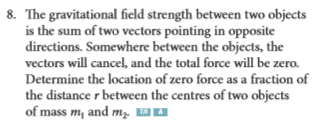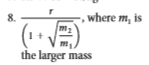# Finding where the gravitational field strength is 0

1. Homework Statementg = (Gm)/r2

## The Attempt at a Solution

I tried doing (Gm1)/a2 = (Gm2)/(r-a)2 where a is equal to the distance from the position we're trying to find to the center of the most massive object but end up getting a quadratic in the denominator on the right side. The answer in the back of the textbook is this:Thank you!

TSny
Homework Helper
Gold Member
I tried doing (Gm1)/a2 = (Gm2)/(r-a)2
Would taking the square root of both sides help? First, you might want to get all of the "squared stuff" on one side of the equation and everything else on the other side.

Would taking the square root of both sides help? First, you might want to get all of the "squared stuff" on one side of the equation and everything else on the other side.
Thanks for your (very) prompt reply! Yeah that helps but now i have (r-a)/a±√(m1/m2). It's very close to the answer but I have no idea what to do with the a :/

TSny
Homework Helper
Gold Member
Thanks for your (very) prompt reply! Yeah that helps but now i have (r-a)/a±√(m1/m2). It's very close to the answer but I have no idea what to do with the a :/
Did you leave out an equals sign somewhere in (r-a)/a±√(m1/m2) ?

Decide whether the + or the - should be used here.

Then, solve for ##a##.

gneill
Mentor
Since you're solving for a fraction of the distance r, why not let a be that fraction so that 0 < a < 1. Then one distance will be ar and the other (1-a)r. Otherwise I think you're going to struggle more than necessary to get the distance differences into fractional form.